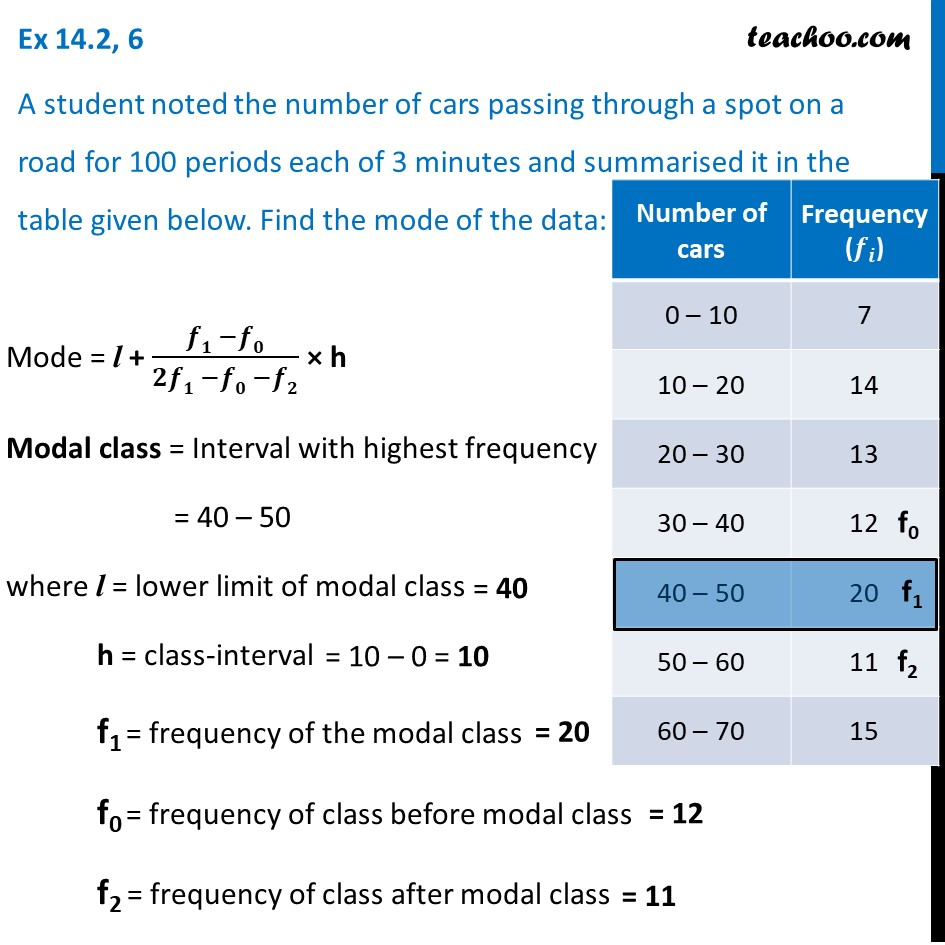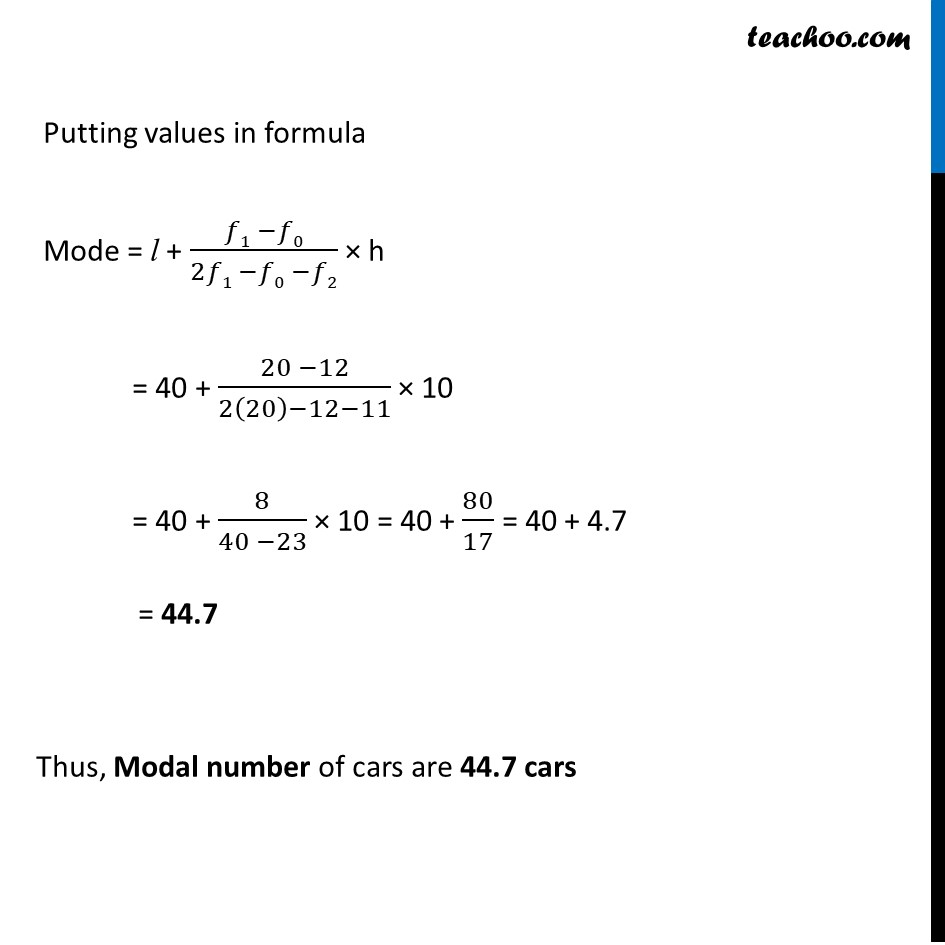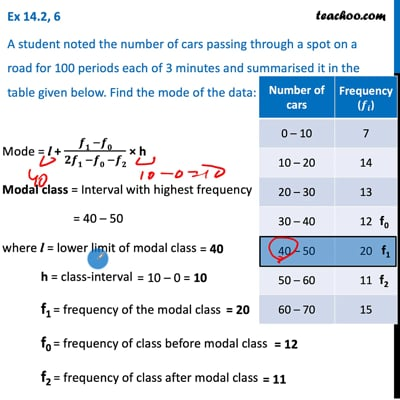Ex 14.2

Chapter 14 Class 10 Statistics
Serial order wiseThis video is only available for Teachoo black users

Solve all your doubts with Teachoo Black (new monthly pack available now!)

### Transcript

Ex 14.2, 6 A student noted the number of cars passing through a spot on a road for 100 periods each of 3 minutes and summarised it in the table given below. Find the mode of the data: Mode = l + (𝒇𝟏 −𝒇𝟎)/(𝟐𝒇𝟏 −𝒇𝟎 −𝒇𝟐) × h Modal class = Interval with highest frequency = 40 – 50 where l = lower limit of modal class h = class-interval f1 = frequency of the modal class f0 = frequency of class before modal class f2 = frequency of class after modal class Putting values in formula Mode = l + (𝑓1 −𝑓0)/(2𝑓1 −𝑓0 −𝑓2) × h = 40 + (20 −12)/(2(20)−12−11) × 10 = 40 + 8/(40 −23) × 10 = 40 + 80/17 = 40 + 4.7 = 44.7 Thus, Modal number of cars are 44.7 cars